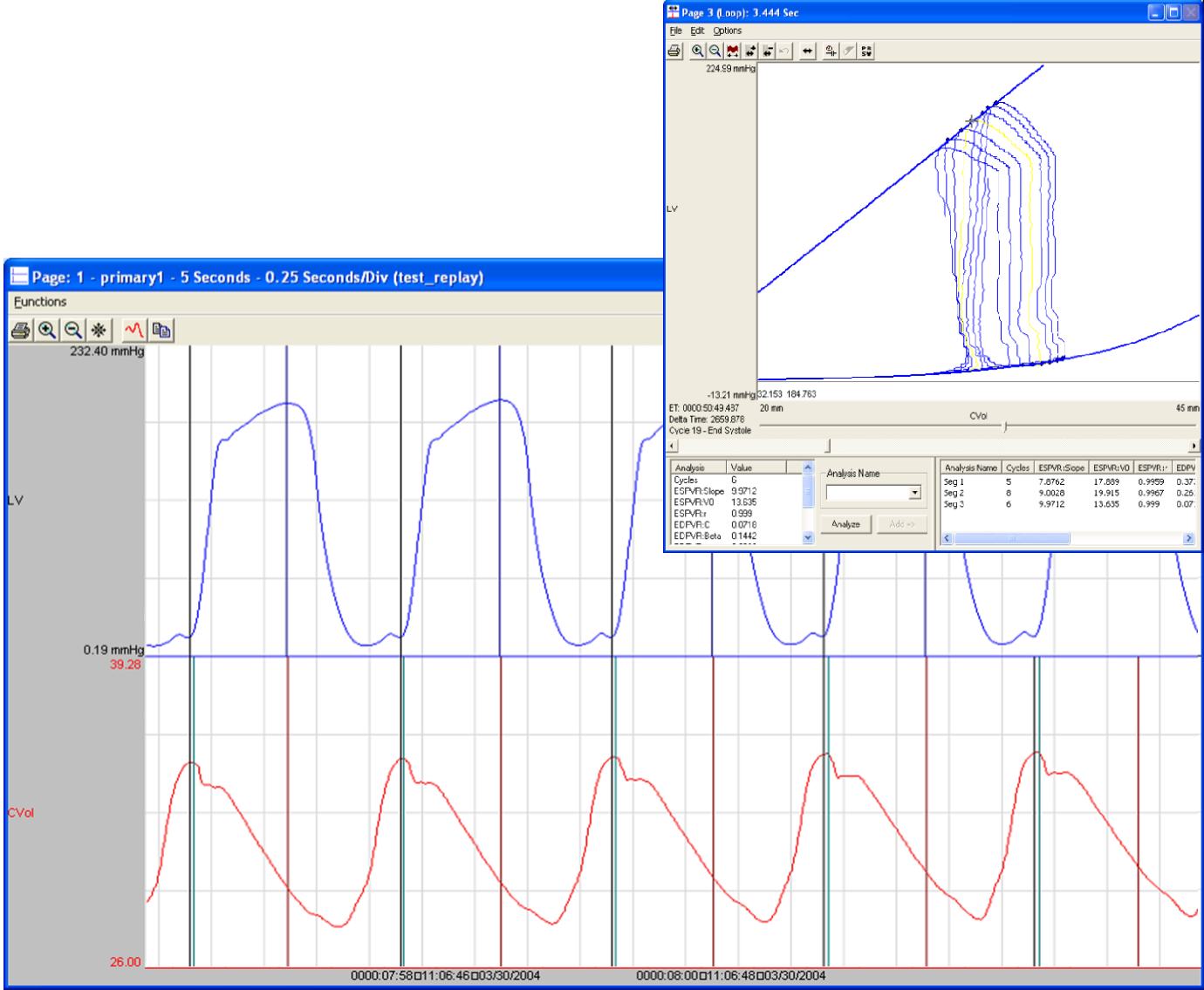# Cardiac Volume Analysis Module

The Cardiac Volume (CVOL) Analysis Module can analyze any volume from the circulatory system and can derive on a beat-to-beat basis values for the cardiac cycle.  Validation marks provide the user with visual documentation that the real-time analysis is providing appropriate cycle recognition.

Additional features built into the Data Review functionality allow the Cardiac Volume channel and LVP channel to be analyzed in a post process mode by the
Pressure-Volume Loop analysis. Pressure-Volume Loops permit the display and analysis of left ventricular pressure cycles plotted against cardiac volume cycles.

The list below describes the parameters calculated by the analysis module either in real-time or during subsequent analysis.

Pressure-Volume Loop analysis features and calculations.

 Cuvette Calibration - Catheter Selection Choose from 40 pre-defined PV catheters for volume calibration during acquisition. Parallel Volume calculation Volume component due to parallel conductance.  Parallel volume is subtracted from summed volume signal by means of a saline bolus injection or input of empirical data. Alpha Correction Factor Reconcile the volume obtained using conductance technology with the volume obtained by other means.  Stroke Volume is divided by Alpha. ESPVR (End Systolic PV Relationship) Slope, X intercept, correlation coefficient. EDPVR (End Diastolic PV Relationship) α, β, X intercept, correlation coefficient. PRSW (Pre Recruitable Stroke Work) Slope, X intercept, correlation coefficient.
##### Model PNM-CVOL100W
 Name Definition Num The number of the cardiac cycle. Vmin The minimum volume value in a cycle. Vmax The maximum volume value in a cycle. SV The stroke volume, which equals Vmax - Vmin. -dPV The volume at the LVP Min Slope mark. Ped The pressure at the LVEDP mark. Ved The volume at the LVEDP mark. %EF Ejection fraction, calculated as Stroke Volume/Vmax * 100. PFR Peak filling rate. -dV Peak emptying rate. TPFR Time to peak filling rate. DFT Diastolic Filling Time. %FT Time to peak filling as a percentage of Diastolic fill time, equals TPFR/DFT * 100. Pes Pressure at max elastance. Ves Volume at max elastance. SW Stroke Work. Ea Arterial elastance. HR Heart rate.  Computed in beats-per-minute. CO Cardiac Output.